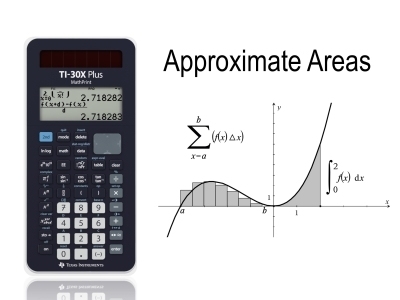# Activities

•• ##### AuthorAust Senior

45 Minutes

## Approximate Areas#### Activity Overview

In this activity students determine the approximate area under a curve using a variety of shapes including squares, rectangles (inner and outer rectangles), triangles and trapezia. An optional extension includes the use of the midpoint and Simpson's rule(s).

This activity includes a video tutorial.

#### Objectives

The purpose of this lesson is to provide students with an understanding of the approximate area under a curve, including how different shapes can be used and that the limiting case leads to integral calculus. The lesson also helps students become very efficient at using the TI-30XPlus MathPrint calculator.

#### Vocabulary

• Inner and Outer Rectangles
• Left and Right Bound Rectangles
• Trapezoidal Rule
• Midpoint Rule (Extension)
• Simpson's Rule (Extension)